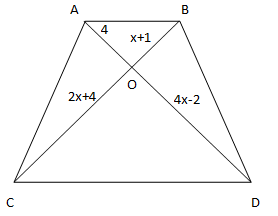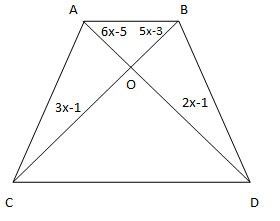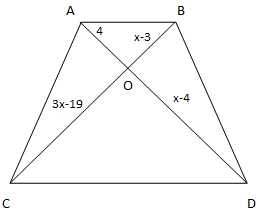GeeksforGeeks App
Open AppBrowser
Continue

## Related Articles

• RD Sharma Class 10 Solutions

# Class 10 RD Sharma Solutions – Chapter 4 Triangles – Exercise 4.4

### Question 1. (i) In fig., if AB || CD, find the value of x.

Solution:Given,

AB∥ CD.

To find the value of x.

Now,

AO/ CO = BO/ DO                         [Diagonals of a parallelogram bisect each other]

⇒ 4/ (4x – 2) = (x +1)/ (2x + 4)

4(2x + 4) = (4x – 2)(x +1)

8x + 16 = x(4x – 2) + 1(4x – 2)

8x + 16 = 4x2 – 2x + 4x – 2

-4x2 + 8x + 16 + 2 – 2x = 0

-4x2 + 6x + 8 = 0

4x2 – 6x – 18 = 0

4x2 – 12x + 6x – 18 = 0

4x(x – 3) + 6(x – 3) = 0

(4x + 6) (x – 3) = 0

∴ x = – 6/4 or x = 3

### (ii) In fig., if AB || CD, find the value of x.

Solution:Given,

AB∥ CD.

To find the value of x.

Now,

AO/ CO = BO/ DO                     [Diagonals of a parallelogram bisect each other]

⇒ (6x – 5)/ (2x + 1) = (5x – 3)/ (3x – 1)

(6x – 5)(3x – 1) = (2x + 1)(5x – 3)

3x(6x – 5) – 1(6x – 5) = 2x(5x – 3) + 1(5x – 3)

18x2 – 10x2 – 21x + 5 + x +3 = 0

8x2 – 20x + 8 = 0

8x2 – 16x – 4x + 8 = 0

8x(x – 2) – 4(x – 2) = 0

(8x – 4)(x – 2) = 0

x = 4/8 = 1/2 or x = -2

∴ x= 1/2

### (iii) In fig., if AB || CD. If OA = 3x – 19, OB = x – 4, OC = x- 3 and OD = 4, find x.

Solution:Given,

AB∥ CD.

OA = 3x – 19, OB = x – 4, OC = x- 3 and OD = 4

To find the value of x.

Now,

AO/ CO = BO/ DO                      [Diagonals of a parallelogram bisect each other]

(3x – 19)/ (x – 3) = (x–4)/ 4

4(3x – 19) = (x – 3) (x – 4)

12x – 76 = x(x – 4) -3(x – 4)

12x – 76 = x2 – 4x – 3x + 12

-x2 + 7x – 12 + 12x -76 = 0

-x2 + 19x – 88 = 0

x2 – 19x + 88 = 0

x2 – 11x – 8x + 88 = 0

x(x – 11) – 8(x – 11) = 0

∴ x = 11 or x = 8

My Personal Notes arrow_drop_up
Related Tutorials